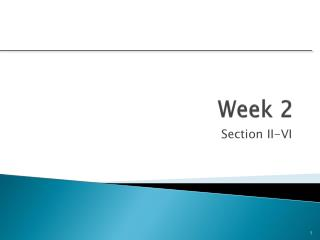DownloadDownload PresentationWeek 2

# Week 2

Télécharger la présentation## Week 2

- - - - - - - - - - - - - - - - - - - - - - - - - - - E N D - - - - - - - - - - - - - - - - - - - - - - - - - - -
##### Presentation Transcript

1. Week 2 Section II-VI

2. II-34

3. RTY chart a.k.a. LeanSigma II-36

4. Financial Models Based on the time value of moneyprincipal – comparing what a dollar is worth today to another time period • Payback period • Net present value • Internal rate of return All of these models use discounted cash flows III-20

5. Time Value of Money • Needs to be accounted for in the capital investment decision • Main reason: capital could be used for something else • Capital has an opportunity cost in any given use III-20

6. Discounted Cash Flow (or DCF) • The discounted cash flow (or DCF) describes a method of valuing a project using the time value of money. • All future cash flows are estimated and discounted to give their present values. • The discount rate reflects two things based on risk: • the time value of money – projecting a future value to today’s dollars. • a cost of capital – a corporate charge for using cash. III-20

7. Payback Period Determines how long it takes for a project to reach a breakeven point Cash flows should be discounted Lower numbers are better(faster payback) III-20

8. Payback Period Example A project requires an initial investment of \$200,000 and will generate cash savings of \$75,000 each year for the next five years. What is the payback period? Divide the cumulative amount by the cash flow amount in the third year and subtract from 3 to find out the moment the project breaks even. III-20

9. Payback Method Advantages/Disadvantages • Advantage • Easy to understand and use • Emphasizes the early recovery of capitol • Cash flow beyond the payback period are uncertain, so they are ignored • Disadvantage • Ignores timing of the cash flow within the payback period • Emphasis is on the recovery of capital, not on profitability • Does not provide a decision criterion for acceptance How do you decide on the maximum allowable payback period? III-20

10. Net Present Value (NPV) • One of the most common project selection metrics. • Predicts the change in the firm’s value if a project is undertaken. • We attempt to equate all cash flows to current dollars Higher NPV values are better! A positive value indicate the firm will make money III-20

11. Discount factor • NPV takes into account a discount factor • The discount factor is simply the reciprocal of the discount rate III-20

12. Net Present Value Example Should you invest \$60,000 in a project that will return \$15,000 per year for five years? You have a minimum return of 8% and expect inflation to hold steady at 3% over the next five years. The NPV column total is negative, so don’t invest! III-20

13. Internal Rate of Return (IRR) • Answers the question: What rate of return will this project earn? • It is the interest rate at which the NPV of the cash flows is equal to zero. • A project must meet a minimum rate of return before it is worthy of consideration. • Need to be solved with MSExcel or a financial based calculator – by hand is an iterative (guessing) process Higher IRR values are better! III-20

14. Internal Rate of Return Example A project that costs \$40,000 will generate cash flows of \$14,000 for the next four years. You have a rate of return requirement of 17%; does this project meet the threshold? This table has been calculated using a discount rate of 15% Actual IRR is 14.9625% The project doesn’t meet our 17% requirement and should not be considered further. III-20

15. Motivation • Definition of motivation on the Web: • the psychological feature that arouses an organism to action toward a desired goal • that which gives purpose and direction to behavior http://wordnetweb.princeton.edu/perl/webwn?s=motivation IV-19

16. Force Field Analysis Example • Create a Force Field Analysis for the following proposal • “Earning a graduate degree after completing your bachelors degree” IV-48